#### the "encoding" of the distribution of prime numbers by the nontrivial zeros of the Riemann zeta function [elegant approach]

The familiar prime counting functionsimply gives the number of primes less than or equal to x. The prime number theorem states that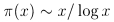. This is already evident in a graph offor x ranging from 0 to 1000: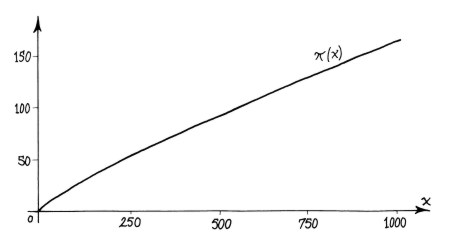This asymptotic relation given by the Prime Number Theorem suggests that there might exist some alternative, logarithmically-weighted prime counting function which is simply asymptotic to x. This turns out to be the case.

Chebyshev introduced the function, which counts not just primes, but also all powers of primes. Each pk for k = 1, 2, 3, ... is counted with weight log p. Formally we write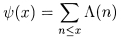Where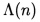are the von Mangoldt values. These vanish for n which are not the power of some prime, and take the value log p when n is a power of prime p: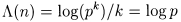It isn't difficult to show the equivalence of the statements. The proof of the Prime Number Theorem usually involves proving the latter.

In many ways,is the more natural counting function. It seems that we should think of primes (and their powers) as having an intrinsic logarithmic weight. In his classic text The Distribution of Prime Numbers, Ingham states:

"It happens that of the three functions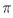,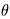,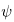, the one which arises most naturaly from the analytical point of view is the one most remote from the original problem, namely. For this reason it is usually most convenient to work in the first instance with...to deduce results about. This is a complication which seems inherent in the subject, and the reader should familiarise himself at the outset with the function, which is to be regarded as the fundamental one."

[Note:is another function introduced by Chebyshev related to the distribution of primes.]

"[medaevil philosopher] William of Occam, elevated to a method the idea that when one must choose between two explanations, one should always choose the simpler. Occam's razor, as the principle is called, cuts out the difficult and chooses the simple. When things get too complicated, it sometimes makes sense to stop and wonder: Have I asked the right question? Here the choice is between two functions that count primes: one is the function psi(x), approximated by a straight line, and the second is pi(x), approximated by the curving function Li(x). Surely William of Occam would have chosen to study psi(x)."
E. Bombieri from "Prime Territory: Exploring the Infinite Landscape at the Base of the Number System" (The Sciences, Sept./Oct. 1992)

Here we see part of the graph of, superimposed over the (asymptotic) function x. If we were to zoom out far enough, the graph of the former would become indistinguishable from the latter.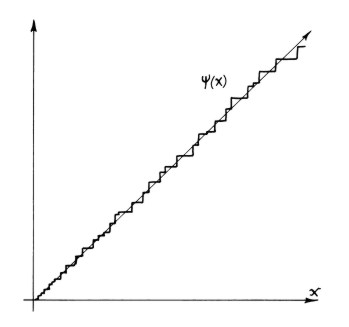It turns out that the (discontinuous) step functioncan be expressed precisely as the limit of a sequence of smooth functions which are based on the complex zeros of the Riemann zeta function.

Directly involved in the usual proof of the Prime Number Theorem is the explicit formula: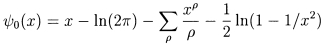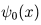differs very slightly fromin that at points of discontinuity it takes a value halfway between its limits on either side, a phenomenon familiar from Fourier analysis.

Note that the fourth term in the right-hand side is neglibible for large x and the second is just a constant.

Therefore, we see that the fluctuations ofabout its asymptotic approximation x are determined by the values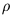, which are the nontrivial zeros of the zeta function, i.e. those with nonzero imaginary parts. These are all known to lie in the critical strip, as illustrated below. It is also known that they must be symmetric about both the real axis and the critical line Re(s) = 1/2.

At present, all known nontrivial zeros lie on the critical line. The Riemann Hypothesis states that they all must (and it is known that there is an infinite number of them). Here are some tables of zeros compiled by Andrew Odlyzko.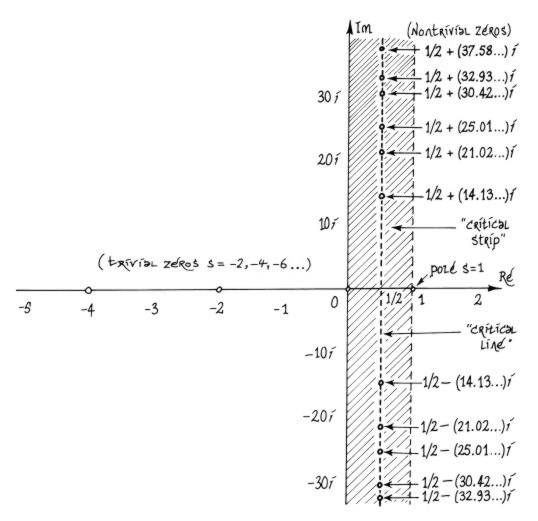Because theare complex, the values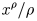must also be complex. However, this is not a problem, as the nontrivial zeros are known to come in complex-conjugate pairsand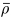. The valuesand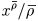are also complex-conjugates, so all imaginary parts cancel in the infinite sum.

Incidentally the function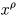maps the positive reals onto a logarithmic spiral in the complex plane.and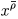produce "complex-conjugate" spirals (mutual reflections across the real axis), so each contribution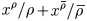=   2Re[]

is a real-valued function, a sort of logarithmically-rescaled sinusoid with increasing amplitude as pictured below: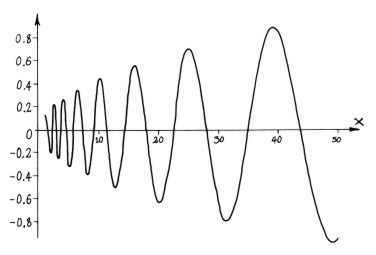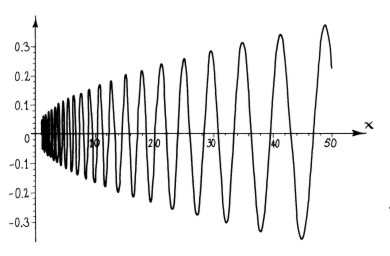Above we see the contributions from the first and sixth nontrivial zeros,= 1/2 + (14.13...)i and= 1/2 + (37.58...)i.

These waveforms are related to the logarithmic spirals in the same way that a normal sine waves are related to the unit circle.

If we were to logarithmically rescale the x-axis, these function would have a constant frequency equal to the imaginary part of. The rate of amplitude growth is related to the real part, which in all known cases is just 1/2.

Returning to the explicit formulawe see that Chebyshev's logarithmic prime counting function can be reconstructed by starting with the function, and then successively adding "spiral wave" functionsgenerated by complex-conjugate pairs,of complex zeta zeros. Below we see an animation where the first 250 pairs of zeros are used to gradually approximate.[animation courtesy of Raymond Manzoni]

Glen Pugh, while at the University of British Columbia, developed a more sophisticated and interactive applet along similar lines. It can be viewed here.

the more common approach
number theory and physics archive      prime numbers: FAQ and resources
mystery      new      search      home